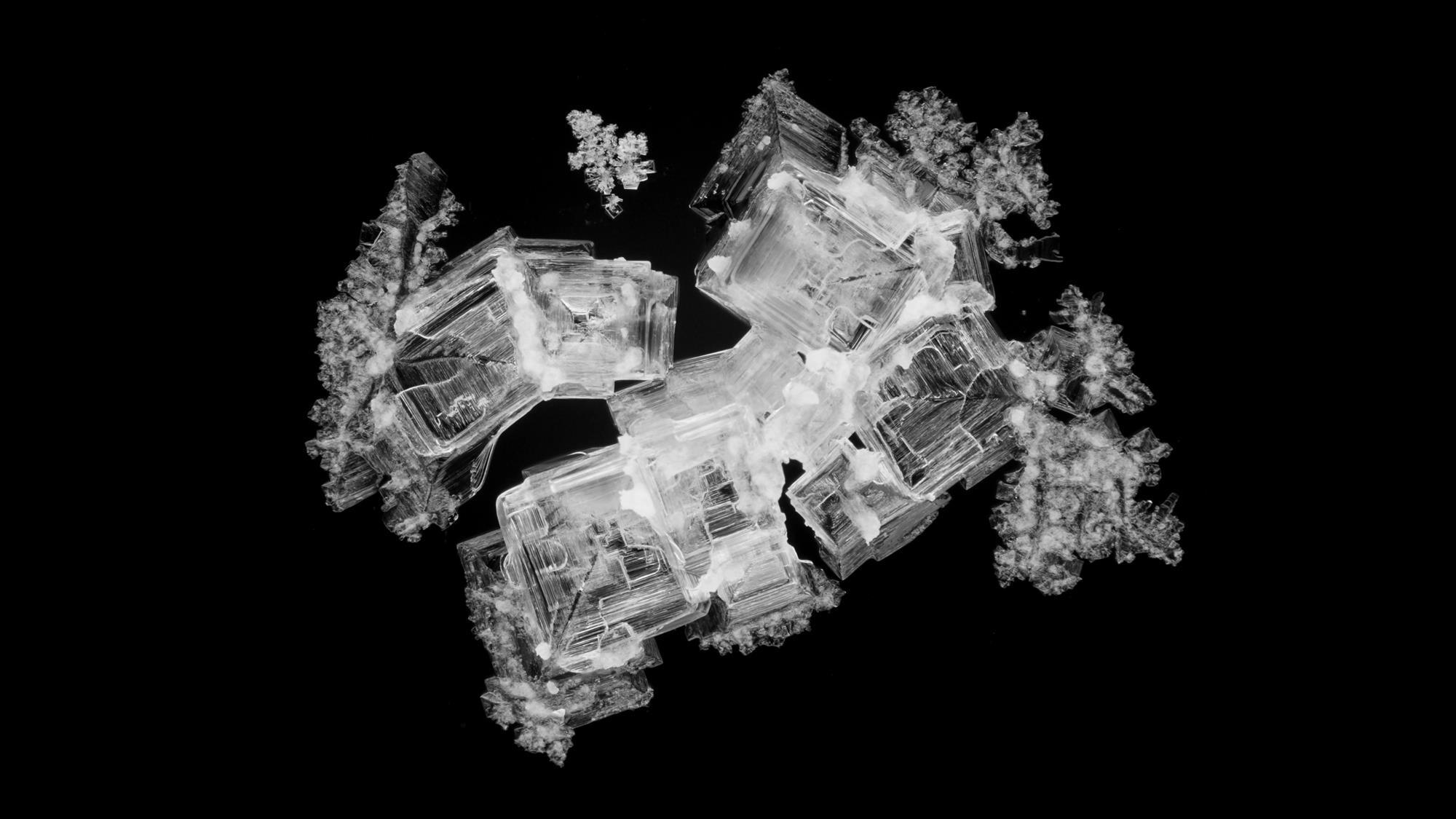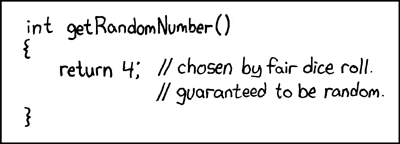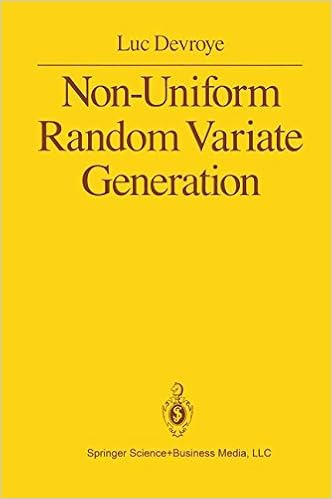## really random generators [again!]

Posted in Books, Statistics with tags , , , , , , , , , on March 2, 2020 by xi'anA pointer sent me to Chemistry World and an article therein about “really random numbers“. Or “truly” random numbers. Or “exactly” random numbers. Not particularly different from the (in)famous lava lamp generator!

“Cronin’s team has developed a robot that can automatically grow crystals in a 10 by 10 array of vials, take photographs of them, and use measurements of their size, orientation, and colour to generate strings of random numbers. The researchers analysed the numbers generated from crystals grown in three solutions – including a solution of copper sulfate – and found that they all passed statistical tests for the quality of their randomness.” Chemistry World, 18 February 2020

The validation of this truly random generator is thus exactly the same as a (“bad”) pseudo-random generator, namely that in the law of large number sense, it fits the predicted behaviour. And thus the difference between them cannot be statistical, but rather cryptographic:

“…we considered the encryption capability of this random number generator versus that of a frequently used pseudorandom number generator, the Mersenne Twister.” Lee et al., Matter, February 10, 2020

Meaning that the knowledge of the starting point and of the deterministic transform for the Mersenne Twister makes it feasible to decipher, which is not the case for a physical and non-reproducible generator as the one advocated. One unclear aspect of the proposed generator is the time required to produce 10⁶, even though the authors mention that “the bit-generation rate is significantly lower than that in other methods”.

## biased sample!

Posted in Statistics with tags , , , , , , , , , , , on May 21, 2019 by xi'anA chance occurrence led me to this thread on R-devel about R sample function generating a bias by taking the integer part of the continuous uniform generator… And then to the note by Kellie Ottoboni and Philip Stark analysing the reason, namely the fact that R uniform [0,1) pseudo-random generator is not perfectly continuously uniform but discrete, by the nature of numbers on a computer. Knuth (1997) showed that in this case the range of probabilities is larger than (1,1), the largest range being (1,1.03). As noted in the note, exploiting directly the pseudo-random bits of the pseudo-random generator. Shocking, isn’t it!  A fast and bias-free alternative suggested by Lemire is available as `dqsample::sample`

As an update of June 2019, sample is now fixed.

## fiducial simulation

Posted in Books, Kids, pictures, Statistics, Travel, University life with tags , , , , , , , , , , , on April 19, 2018 by xi'anWhile reading Confidence, Likelihood, Probability), by Tore Schweder and Nils Hjort, in the train from Oxford to Warwick, I came upon this unexpected property shown by Lindqvist and Taraldsen (Biometrika, 2005) that to simulate a sample y conditional on the realisation of a sufficient statistic, T(y)=t⁰, it is sufficient (!!!) to simulate the components of  y as y=G(u,θ), with u a random variable with fixed distribution, e.g., a U(0,1), and to solve in θ the fixed point equation T(y)=t⁰. Assuming there exists a single solution. Brilliant (like an aurora borealis)! To borrow a simple example from the authors, take an exponential sample to be simulated given the sum statistics. As it is well-known, the conditional distribution is then a (rescaled) Beta and the proposed algorithm ends up being a standard Beta generator. For the method to work in general, T(y) must factorise through a function of the u’s, a so-called pivotal condition which brings us back to my post title. If this condition does not hold, the authors once again brilliantly introduce a pseudo-prior distribution on the parameter θ to make it independent from the u’s conditional on T(y)=t⁰. And discuss the choice of the Jeffreys prior as optimal in this setting even when this prior is improper. While the setting is necessarily one of exponential families and of sufficient conditioning statistics, I find it amazing that this property is not more well-known [at least by me!]. And wonder if there is an equivalent outside exponential families, for instance for simulating a t sample conditional on the average of this sample.

## a null hypothesis with a 99% probability to be true…

Posted in Books, R, Statistics, University life with tags , , , , , , , , , , , on March 28, 2018 by xi'anWhen checking the Python t distribution random generator, np.random.standard_t(), I came upon this manual page, which actually does not explain how the random generator works but spends instead the whole page to recall Gosset’s t test, illustrating its use on an energy intake of 11 women, but ending up misleading the readers by interpreting a .009 one-sided p-value as meaning “the null hypothesis [on the hypothesised mean] has a probability of about 99% of being true”! Actually, Python’s standard deviation estimator x.std() further returns by default a non-standard standard deviation, dividing by n rather than n-1…## complexity of the von Neumann algorithm

Posted in Statistics with tags , , , , , , , , , on April 3, 2017 by xi'an“Without the possibility of computing infimum and supremum of the density f over compact subintervals of the domain of f, sampling absolutely continuous distribution using the rejection method seems to be impossible in total generality.”

The von Neumann algorithm is another name for the rejection method introduced by von Neumann circa 1951. It was thus most exciting to spot a paper by Luc Devroye and Claude Gravel appearing in the latest Statistics and Computing. Assessing the method in terms of random bits and precision. Specifically, assuming that the only available random generator is one of random bits, which necessarily leads to an approximation when the target is a continuous density. The authors first propose a bisection algorithm for distributions defined on a compact interval, which compares random bits with recursive bisections of the unit interval and stops when the interval is small enough.In higher dimension, for densities f over the unit hypercube, they recall that the original algorithm consisted in simulating uniforms x and u over the hypercube and [0,1], using the uniform as the proposal distribution and comparing the density at x, f(x), with the rescaled uniform. When using only random bits, the proposed method is based on a quadtree that subdivides the unit hypercube into smaller and smaller hypercubes until the selected hypercube is entirely above or below the density. And is small enough for the desired precision. This obviously requires for the computation of the upper and lower bound of the density over the hypercubes to be feasible, with Devroye and Gravel considering that this is a necessary property as shown by the above quote. Densities with non-compact support can be re-expressed as densities on the unit hypercube thanks to the cdf transform. (Actually, this is equivalent to the general accept-reject algorithm, based on the associated proposal.)

“With the oracles introduced in our modification of von Neumann’s method, we believe that it is impossible to design a rejection algorithm for densities that are not Riemann-integrable, so the question of the design of a universally valid rejection algorithm under the random bit model remains open.”

In conclusion, I enjoyed very much reading this paper, especially the reflection it proposes on the connection between Riemann integrability and rejection algorithms. (Actually, I cannot think straight away of a simulation algorithm that would handle non-Riemann-integrable densities, apart from nested sampling. Or of significant non-Riemann-integrable densities.)

## ratio-of-uniforms [-1]

Posted in Books, pictures, R, Statistics, University life with tags , , , on December 12, 2016 by xi'anLuca Martino pointed out to me my own and forgotten review of a 2012 paper of his, “On the Generalized Ratio of Uniforms as a Combination of Transformed Rejection and Extended Inverse of Density Sampling” that obviously discusses a generalised version of Kinderman and Monahan’s (1977) ratio-of-uniform method. And further points out the earlier 1991 paper by Jon Wakefield, Alan Gelfand and Adrian Smith that contains the general form I rediscovered a few posts ago… Called the GRoU in Martino et al.. While the generalisation in the massive arXiv document is in finding Φ such that the above region is bounded and can be explored by uniform sampling over a box.

Neither reference mentions using the cdf transform, though, which does guarantee a bounded ratio-of-uniform set in u. (An apparent contradiction with Martino et al.  statement (34), unless I am confused. Maybe due to using Φ⁻¹ instead of Φ?) But I still wonder at the usefulness of my derivations those past weeks!

## Sobol’s Monte Carlo

Posted in Books, pictures, Statistics, University life with tags , , , , , , , , , , on December 10, 2016 by xi'anThe name of Ilya Sobol is familiar to researchers in quasi-Monte Carlo methods for his Sobol’s sequences. I was thus surprised to find in my office a small book entitled The Monte Carlo Method by this author, which is a translation of his 1968 book in Russian. I have no idea how it reached my office and I went to check with the library of Paris-Dauphine around the corner [of my corridor] whether it had been lost: apparently, the library got rid of it among a collection of old books… Now, having read through this 67 pages book (or booklet as Sobol puts it) makes me somewhat agree with the librarians, in that there is nothing of major relevance in this short introduction. It is quite interesting to go through the book and see the basics of simulation principles and Monte Carlo techniques unfolding, from the inverse cdf principle [established by a rather convoluted proof] to importance sampling, but the amount of information is about equivalent to the Wikipedia entry on the topic. From an historical perspective, it is also captivating to see the efforts to connect physical random generators (such as those based on vacuum tube noise) to shift-register pseudo-random generators created by Sobol in 1958. On a Soviet Strela computer.

While Googling the title of that book could not provide any connection, I found out that a 1994 version had been published under the title of A Primer for the Monte Carlo Method, which is mostly the same as my version, except for a few additional sections on pseudo-random generation, from the congruential method (with a FORTRAN code) to the accept-reject method being then called von Neumann’s instead of Neyman’s, to the notion of constructive dimension of a simulation technique, which amounts to demarginalisation, to quasi-Monte Carlo [for three pages]. A funny side note is that the author notes in the preface that the first translation [now in my office] was published without his permission!